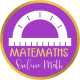Sphere

sphere is the set of all points, in three-dimensional space, which are equidistant from a point. The radius has one endpoint on the sphere and the other endpoint at the center of that sphere. The diameter of a sphere must contain the center and touch two points of the sphere.The largest circular cross-section in a sphere is a great circle. The circumference of a sphere is the circumference of a great circle. Every great circle divides a sphere into two congruent hemispheres (half a sphere).Surface area of a sphere

Surface area of a sphere is the two-dimensional measurement that includes the total area of all surfaces that bound the sphere. The basic unit of area is the square unit.

The formula to calculate the total surface of a sphere is:Inversae formula:Volume of a sphere

To find the volume of a sphere you must figure out how much space it occupies. The basic unit of volume is the cubic unit.

The formula to calculate the volume of a sphere is:Inversae formulaExercises

1. Find the surface area of the sphere whose radius is 4 cm.
2. What is the diameter of the sphere, if the surface area of the sphere is 576π cm2.
3. What is the diameter of the sphere, if the surface area of the sphere is 784π cm2.
4. The volume of a sphere divided by its surface area is 9 cm. What is the radius of the sphere?
5. The volume of a sphere divided by its surface area is 7 cm. What is the radius of the sphere?
6. What is the volume of the hemisphere, if the diameter of the hemisphere is 7.2 cm.
7. Find the surface area of the earth assuming the earth to be a sphere of radius 6369 km.
8. Find the sum of the volume of a cone with radius 1 cm and height 5 cm and the volume of a sphere of radius 1 cm.
9. An adhesive compound in liquid form is prepared in a container of hemispherical shape having a radius of 180 cm.This compound is to be packed in cylindrical bottles of radius 1 cm and height of 4 cm. How many bottles are needed if the liquid prepared exactly fills the container?

3 thoughts on “Sphere”

1.Alberto Damiano says:

We need to see the solutions!

Like

2.Alberto Damiano says:

We want to see the solutions! Also, a little math gem: the surface area is the derivative of the volume with respect to r. True for all spheres in any dimension, including the circle!

Like

1.matemaths says:

Give me time and you’ll see the solutions

Like

This site uses Akismet to reduce spam. Learn how your comment data is processed.